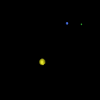# Dynamical systems: Simulation and visualization

One of the goals of the dynamical systems project at the Geometry Center is an interface between the software package DsTool, for numerical simulation of dynamical systems (created at the Center for Applied Mathematics, Cornell University), and Geomview, for visualization of geometric objects in 3 (and higher) dimensions (created at the Geometry Center). This interface allows one to see, as it is computed, a trajectory in configuration space of the dynamical system being modelled.

Several examples are given below and short movies have been made of a specific computed trajectory.

Also, two students from the 1996 summer program working with Bob Thurman and Patrick Worfolk (postdocs at the Geometry Center) worked on computing and visualizing halo orbits and their stable and unstable manifolds for the restricted three body problem.

### The double pendulumA double pendulum is one pendulum hung from a second pendulum. Initial angular velocities are given, but then the pendulum swings freely under the influence of gravity. The resulting dynamics can be quite remarkable. One trajectory may be viewed as an mpeg movie (360K).

### The restricted three body problemThe restricted three body problem is a model for the motion of a spacecraft moving under the gravitational influence of two larger bodies which are prescribing circular orbits around each other. For example, this models a spacecraft in the earth-moon system; or a body moving in the Sun-Jupiter system. A short mpeg movie (90K) is available which shows one particular orbit in such a system.

### An oscillating surfaceFinite dimensional dynamical systems are often used to approximate PDE problems for the evolution of some sort of surface or interface. Here we demonstrate in an mpeg movie (385K) a simulation of the motion of such a surface whose motion is specified by a 2-dimensional dynamical system.

The DsTool software with a brand new (as of 1997!) interface written in Tcl/Tk and running on Unix workstations and PCs with linux is available from this site at Cornell:
http://www.math.cornell.edu/~gucken/software.html
Up: Geometry Center Projects